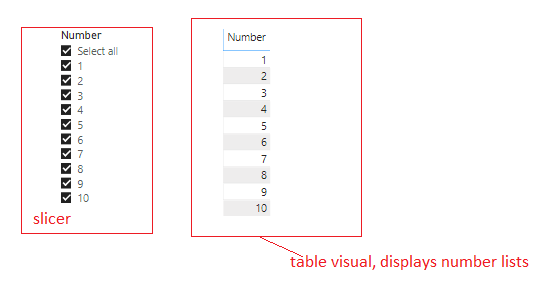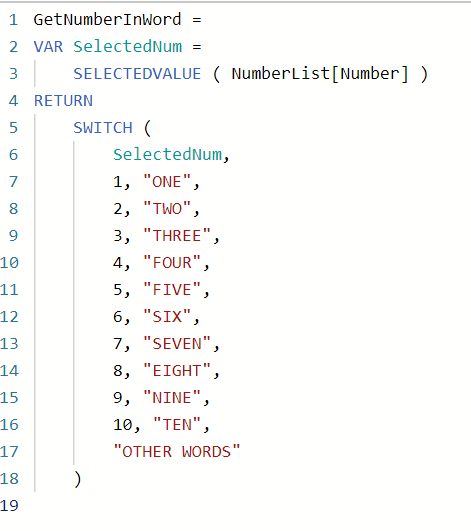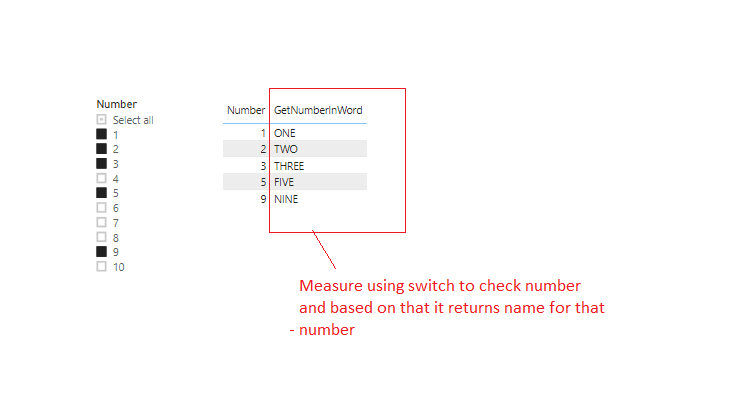Home » SWITCH FUNCTION DAX

# SWITCH FUNCTION DAXSWITCH Function is a Power BI DAX logical function which evaluates an expression against a list of values and returns different results depending on the value of an expression.

It returns a scalar value of any type.

Scalar value that either coming from one of the result expression it is matched with value or from else expression if it is not matched with any value.

DAX SYNTAX

`SWITCH(<expression>, <value>, <result>[, <value>, <result>]…[, <else>])`

<expression> is an expression that is to be evaluated, returns scalar value.

<value> is constant value that is to be matched with the results of an expression.

<result> is the result to be returned if expression has corresponding value.

<else> If there are no matching values the Else value is returned. It is an optional. Else expression must have same data type as result expression.

Lets go through an example to see SWITCH Function in action.

As you can see, here we have a slicer which contains number lists from 1 to 10 and a table visual which displays those numbers.Now we have to display a name against each number in table visual means if number is 1 then it will be “one”, if 2 then “two” and so on..

For this we will create a DAX using switch function which evaluates an expression against a list of numbers and returns different results.

```GetNumberInWord =

VAR SelectedNum =

SELECTEDVALUE ( NumberList[Number] )

RETURN

SWITCH (

SelectedNum,

1, "ONE",

2, "TWO",

3, "THREE",

4, "FOUR",

5, "FIVE",

6, "SIX",

7, "SEVEN",

8, "EIGHT",

9, "NINE",

10, "TEN",

"OTHER WORDS"

)```Once you create the measure, lets drag the measure into table visual next to Number field.
As you can see for selected numbers in slicers, it returns a name of selected numbers.